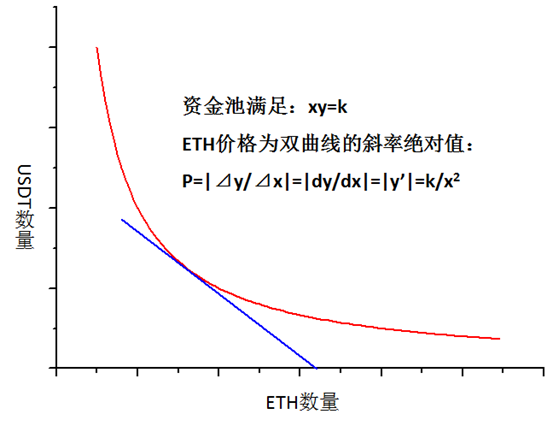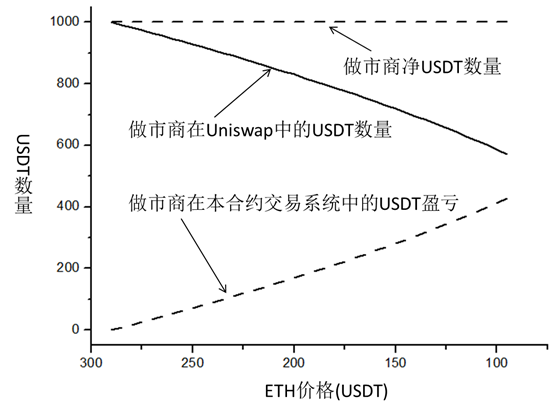# 一种可完全对冲无常损失和单一资产挖矿的合约产品

xy=k

y=k/xx0=(k/P0)^0.5

xn=(k/Pn)^0.5

y0=(kP0)^0.5

yn=(kPn)^0.5

yn=y0*(Pn/P0)^0.51、流动性对冲用户 2、币本位看涨用户 3、价差套利用户 4、吃费率用户